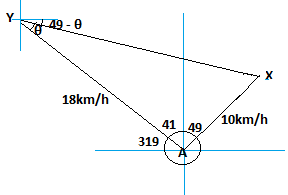mycollegehive
Maths
Math0Two cyclist X and Y leave town A at the same time. Cyclist X travels at the rate of 10km per hour on a bearing of 049degrees and cyclist Y travels at the rate of 18km on a bearing of 319degrees calculate  (a) bearing of cyclist X from Y (b)find the average speed at which cyclist X will get to Y in 4years.

34 viewsShareFollowNigeria
13 September 2020Joshua43,
Yes it's in km/h
0 likes, 0 dislikesplease check the question properly... is it 18km or 18km per hour
0 likes, 0 dislikesSchool not set Nigeria
14 September 20200opp = 10

tanθ = 10/18

tanθ = 0.8

θ = tan-1(0.8)

θ = 29.05

Therefore, the bearing of x from y is 90 + 49 - θ

= 180 + 49 - 29.05

= 109.95

b) from pyhtagoras theorem, hyp2 = opp2 + adj2

hyp = XY

opp = 18

XY2 = 182 + 102

XY = √(182 + 102)

XY = 20.59

speed of XY = distance/time

time = 4 years = 35040 hours

speed of XY = 20.59/35040

= 0.00059km/h OR 5.1475km/yearShare### Related Tags

Math

9 followers

1018 questions